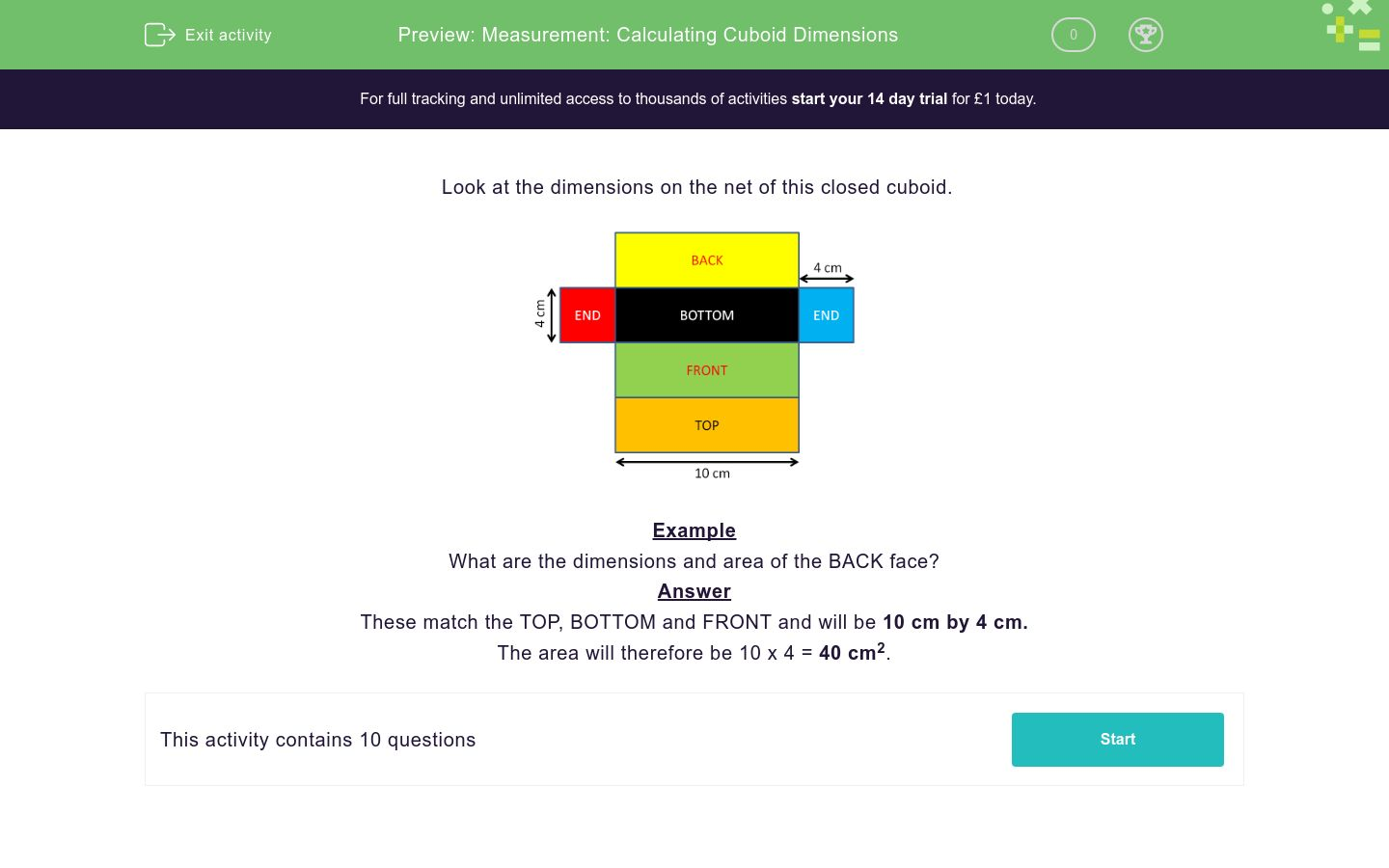# Measurement: Calculating Cuboid Dimensions

In this worksheet, students answer questions on the net of a cuboid.Key stage:  KS 2

Curriculum topic:   Measurement

Curriculum subtopic:   Use Cubes/Cuboids

Difficulty level:### QUESTION 1 of 10

Look at the dimensions on the net of this closed cuboid.Example

What are the dimensions and area of the BACK face?

These match the TOP, BOTTOM and FRONT and will be 10 cm by 4 cm.

The area will therefore be 10 x 4 = 40 cm2.

Look at the net of this closed cuboid.

What is the area of the BOTTOM face in cm2?

(Just write the number)Look at the net of this closed cuboid.

What is the area of one END face in cm2?

(Just write the number)Look at the net of this closed cuboid.

What is the surface area of the whole cuboid in cm2?

(Just write the number)Look at the net of this closed cuboid.

What is the volume of the whole cuboid in cm3?

(Just write the number)Look at the net of this closed cuboid.

What is the area of the BOTTOM face in cm2?

(Just write the number)Look at the net of this closed cuboid.

What is the area of the BACK face in cm2?

(Just write the number)Look at the net of this closed cuboid.

What is the area of the TOP face in cm2?

(Just write the number)Look at the net of this closed cuboid.

What is the area of an END face in cm2?

(Just write the number)Look at the net of this closed cuboid.

What is the SURFACE AREA of the cuboid in cm2?

(Just write the number)Look at the net of this closed cuboid.

What is the VOLUME of the cuboid in cm3?

(Just write the number)• Question 1

Look at the net of this closed cuboid.

What is the area of the BOTTOM face in cm2?

(Just write the number)40
EDDIE SAYS
10 x 4 = 40
• Question 2

Look at the net of this closed cuboid.

What is the area of one END face in cm2?

(Just write the number)16
EDDIE SAYS
4 x 4 = 16
• Question 3

Look at the net of this closed cuboid.

What is the surface area of the whole cuboid in cm2?

(Just write the number)192
EDDIE SAYS
4 x 40 + 2 x 16 = 160 + 32 = 192
• Question 4

Look at the net of this closed cuboid.

What is the volume of the whole cuboid in cm3?

(Just write the number)160
EDDIE SAYS
4 x 4 x 10 = 160
• Question 5

Look at the net of this closed cuboid.

What is the area of the BOTTOM face in cm2?

(Just write the number)40
EDDIE SAYS
10 x 4 = 40
• Question 6

Look at the net of this closed cuboid.

What is the area of the BACK face in cm2?

(Just write the number)70
EDDIE SAYS
10 x 7 = 70
• Question 7

Look at the net of this closed cuboid.

What is the area of the TOP face in cm2?

(Just write the number)40
EDDIE SAYS
10 x 4 = 40
• Question 8

Look at the net of this closed cuboid.

What is the area of an END face in cm2?

(Just write the number)28
EDDIE SAYS
4 x 7 = 28
• Question 9

Look at the net of this closed cuboid.

What is the SURFACE AREA of the cuboid in cm2?

(Just write the number)276
EDDIE SAYS
70 + 70 + 40 + 40 + 28 + 28 = 276
• Question 10

Look at the net of this closed cuboid.

What is the VOLUME of the cuboid in cm3?

(Just write the number)280
EDDIE SAYS
4 x 7 x 10 = 280
---- OR ----

Sign up for a £1 trial so you can track and measure your child's progress on this activity.

### What is EdPlace?

We're your National Curriculum aligned online education content provider helping each child succeed in English, maths and science from year 1 to GCSE. With an EdPlace account you’ll be able to track and measure progress, helping each child achieve their best. We build confidence and attainment by personalising each child’s learning at a level that suits them.

Get started